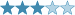## Cycle Killer - Q'est-ce que c'est?

(Eingestellt am 15. September 2021, 19:49 Uhr von SirSchmoopy)

This is my second puzzle working with this concept of 'cycles' and probably my favorite puzzle that I've constructed (though I'm still relatively new so that's not saying much). This should be a bit trickier than my first cycle puzzle, though still relatively approachableStandard Rules:

• Normal Sudoku rules apply.
• Normal Little Killer rules apply. Digits along diagonals pointed to by arrows outside the grid must sum to the indicated total.

Cycle Definitions:

1. Let a horizontal cycle be defined as follows:

• For a digit A in column Z, look to the digit in the Ath Column.
• For this digit B in the Ath column, look to the digit in the Bth column.
• For this digit C in the Bth column, look to the digit in the Cth column...
• Repeat this process until you are back to the digit A (i.e. - eventually you will get to column Y which contains Z as its digit, and this points back to the Zth column where you started with the digit A).
The horizontal cycle is then (A -> B -> C -> ... -> Y -> Z)

2. Let the order of a cycle be the number of unique digits contained in that cycle. (for a concreate example, if a cycle is (3 -> 6 ), with 6 pointing back to 3, that cycle would have an order of 2)

Cycle Killer Rules:

• In addition to standard little killer rules, the sum of the order of the horizontal cycles of the cells along the diagonal of a little killer clue also adds to the number of the clue. (e.g. The digits of R1C8 and R2C9 sum to 8. Furthermore, the order of R1C8's cycle plus the order of R2C9's cycle sums to 8)
• Shaded cells have a digit equal to its horizontal cycle's order. (e.g. if a shaded cell's digit was 3, that cell would be part of an order 3 horizontal cycle). No negative constraint applies.

Cycle Examples:

Shaded cells below all have a horizontal cycle with order equal to their digit. I've illustrated the path the cycle takes, as well as numbered the order of the path assuming the 'start' of the cycle is the shaded cell (note that the 'start' of a cycle is completely arbitrary, and has no impact on the cycle's order)

In the second example, you can see a valid cycle killer clue. Both digits along the little killer clue are part of order-2 cycles, and 2+2 = 1+3 = 4.Lösungscode: The Digits in row 4 (left to right) and the Digits in column 5 (top to bottom) with no spaces (e.g. 123456789123456789)

Zuletzt geändert am 16. September 2021, 16:17 Uhr

Gelöst von Maxima, PrimeWeasel, Zombie Hunter, twobear
Komplette Liste

### Kommentare

am 29. September 2021, 12:42 Uhr von Hausigel_mod
Labels changed

am 28. September 2021, 06:44 Uhr von twobear
Very nice construction. Thank you!

am 16. September 2021, 16:16 Uhr von SirSchmoopy
added a valid cycle killer example, thanks for the feedback PrimeWeasel!

am 16. September 2021, 15:31 Uhr von PrimeWeasel
Very well constructed! I think the addendum to the Little killer might need an example or some rephrasing cause it took me a while to understand what you meant, but other than that, great puzzle!

 Schwierigkeit:Bewertung: N/A Gelöst: 4 mal Beobachtet: 0 mal ID: 0007LM

Lösungscode:

## Anmelden